### Home > CALC > Chapter 5 > Lesson 5.1.4 > Problem5-40

5-40.
1. A rectangle is bounded by the function y = −x2 + 5 and the x-axis as shown below. Homework Help ✎

2.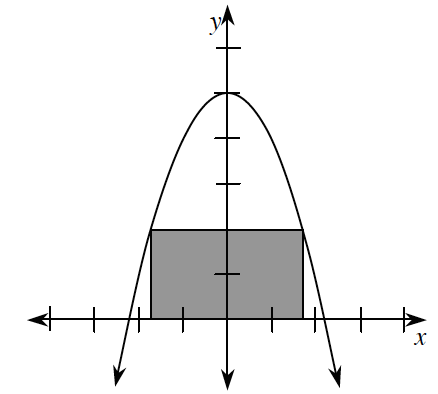1. If the base of the rectangle is 2x, what is the height?

2. What is the maximum area that the rectangle can obtain?

3. If you made the rectangle from part (b) into a flag and spun it around the x-axis, what would be the resulting volume?

4. Find x so that the volume of the rotated rectangle is a maximum.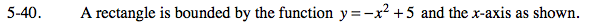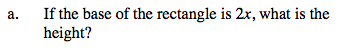Notice that the rectangle is symmetric across the y-axis.

Because of the symmetry, the endpoints of the rectangle can be found at −x and +x... after all, the distance between −x and x is 2x, the length of the base.

Consequently, the height of the rectangle can be evaluated at f(x):
height = y = −x2 + 5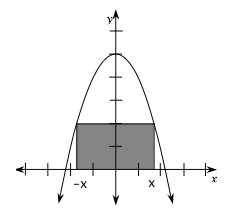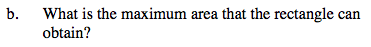Area = (base)(height)
Use the base and height you found in part (a).Then optimize the Area function.

To find the maximum Area:
1. Let A'(x) = 0.
2. Solve for x.
3. Use the x and your expressions for base & height to calculate the maximum area.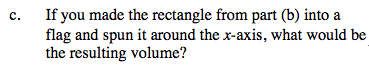A cylinder with height __________ and radius __________.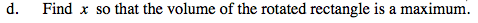Volume of a cylinder: V = πr2h
If you need guidance optimizing the volume, refer to steps in part (b).